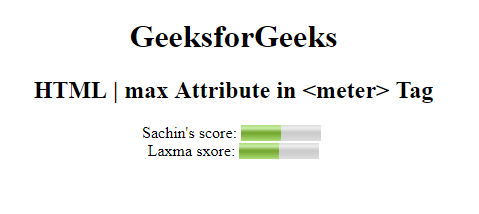# HTML | <meter> max Attribute

The max Attribute in <meter> element is used to specify the upper bound of the gauge. The value of the max attribute must be greater than the min attribute. It has a default value which is 1.

Syntax:

`<meter max="number">`

Attribute Values: It contains the value i.e number which specifies the maximum value of the gauge.

Example: this Example illustrates the use of max attribute in meter Element.

## html

 `` `<``html``>` `<``head``>` `    ``<``title``>` `        ``HTML | max attribute` `    ``` ``   `<``body` `style="text-align:center;">` `    ``<``h1``> ` `      ``GeeksforGeeks ` `  ```   `    ``<``h2``> ` `      ``HTML | max Attribute in  ` `      ``<meter> Tag `   `  `` Sachin's score:`   `    ``<``meter` `value="5" ` `           ``min="0"` `           ``max="10" ` `           ``high="6">` `        ``5 out of 10` `    ```   `    ``<``br``>Laxma sxore:` `    ``<``meter` `value="0.5"` `           ``max="1.0" ` `           ``min="0"` `           ``high="0.6">` `        ``50% from 100%` `    ```   ``   ``

Output:Supported Browsers: The browser supported by HTML | <meter>max Attribute are listed below: